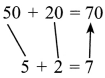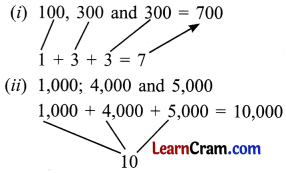# DAV Class 4 Maths Chapter 2 Worksheet 3 Solutions

The DAV Class 4 Maths Book Solutions and DAV Class 4 Maths Chapter 2 Worksheet 3 Solutions of Addition and Subtraction offer comprehensive answers to textbook questions.

## DAV Class 4 Maths Ch 2 WS 3 Solutions

Question 1.
(a) 70 + 20 = ___________
90

(b) 30 + 40 = ___________
70

(c) 20 + 60 + 10 = ___________
90(d) 30 + 20 + 30 = ___________
80

(e) 30 + 40 + 20 = ___________
90

(f) 700 + 200 = ___________
900

(g) 900 + 500 = ___________
1,400

(h) 100 + 200 + 500 = ___________
800

(i) 200 + 300 + 200 = ___________
700

(j) 400 + 300 + 100 = ___________
800

(k) 2,000 + 3,000 = ___________
5,000

(l) 5,000 + 11,0 00 + 2,000 = ___________
18,000

(m) 13,000 + 3,000 + 13,000 = ___________
29,000(n) 47,000 + 13,000 = ___________